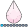NKormanik
Barite | Level 11

## Two Time Series -- Measure of 'In-Sync' or 'In-Phase'

Suppose we have two time-series, X1 and X2.  I'm wondering if SAS has a way of showing if the two series are in-sync, or in-phase, vs. out of sync/phase.

Let's say we want to execute some action when the sync of X1, X2 is maximally moving in the same direction.

We have to be able to track the sync/phase.  Have a measure thereof.

Seems what I am seeking is like a 'moving correlation' -- which takes into account the previous so many days of data.

Any thoughts greatly appreciated.

Nicholas Kormanik

2 REPLIES 2

Nicholas,

Best,

K

## Re: Two Time Series -- Measure of 'In-Sync' or 'In-Phase'

I've always been disappointed that PROC EXPAND only does univariate series transformations.  That's why in the useful paper mentioned by @KatScott you have to prepare the dataset (e.g. create product X1_2=x1*x2) prior to PROC EXPAND, which of course is followed by a PROC CORR.

It might be a better idea to skip the proc expand, as in:

``````
data need (drop=_:) view=need ;
set have;
if _n_=1 then do;
declare hash h (dataset:'have(obs=0)',ordered:'a');
h.definekey('date');
h.definedata(all:'Y');
h.definedone();
declare hiter hi ('h');
end;

_date&winsize=lag&winsize(date);
window_close_date=date ;
format window_close_date date9. ;

if _date&winsize^=. then h.remove(key:_date&winsize);

if _n_>=&winsize then do _rc=hi.first() by 0 until (hi.next()^=0);
output;
end;
run;

proc corr data=need noprint  out=correlations;
by window_close_date ;
var x y;
run;``````

Dataset view NEED simply creates a set of records for each window.  In the above instance the window size is 21, and is identified by the variable WINDOW_CLOSE_DATE.  Each WINDOW_SIZE_DATE will have 21 records ( and therefore most records will appear in 21 windows) in NEED.   For a large windows size, that's a lot of records, but disk activity is kept to a minimum by generating NEED as a data set VIEW, not a data set FILE.

--------------------------
The hash OUTPUT method will overwrite a SAS data set, but not append. That can be costly. Consider voting for Add a HASH object method which would append a hash object to an existing SAS data set

Would enabling PROC SORT to simultaneously output multiple datasets be useful? Then vote for
Allow PROC SORT to output multiple datasets

--------------------------
Discussion stats
• 2 replies
• 219 views
• 1 like
• 3 in conversation